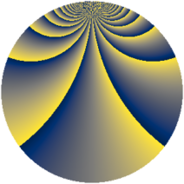# Properties

 Label 1323.2.wLevel $1323$ Weight $2$ Character orbit 1323.w Rep. character $\chi_{1323}(148,\cdot)$ Character field $\Q(\zeta_{9})$ Dimension $708$ Sturm bound $336$

# Related objects

## Defining parameters

 Level: $$N$$ $$=$$ $$1323 = 3^{3} \cdot 7^{2}$$ Weight: $$k$$ $$=$$ $$2$$ Character orbit: $$[\chi]$$ $$=$$ 1323.w (of order $$9$$ and degree $$6$$) Character conductor: $$\operatorname{cond}(\chi)$$ $$=$$ $$27$$ Character field: $$\Q(\zeta_{9})$$ Sturm bound: $$336$$

## Dimensions

The following table gives the dimensions of various subspaces of $$M_{2}(1323, [\chi])$$.

Total New Old
Modular forms 1056 768 288
Cusp forms 960 708 252
Eisenstein series 96 60 36

## Trace form

 $$708q + 6q^{2} + 6q^{3} + 6q^{4} + 9q^{5} - 6q^{8} + O(q^{10})$$ $$708q + 6q^{2} + 6q^{3} + 6q^{4} + 9q^{5} - 6q^{8} + 3q^{10} + 3q^{11} + 24q^{12} + 6q^{13} - 39q^{15} - 9q^{17} + 21q^{18} + 3q^{19} - 3q^{20} - 21q^{22} + 12q^{23} - 54q^{24} + 15q^{25} + 66q^{26} + 3q^{27} - 66q^{29} + 111q^{30} + 15q^{31} - 72q^{32} + 48q^{33} + 9q^{34} - 102q^{36} + 3q^{37} - 18q^{38} - 33q^{39} - 21q^{40} + 51q^{41} - 21q^{43} + 9q^{44} - 33q^{45} + 3q^{46} + 69q^{47} + 93q^{48} - 159q^{50} + 12q^{51} + 45q^{52} - 54q^{53} + 90q^{54} + 12q^{55} - 87q^{57} + 33q^{58} - 30q^{59} - 156q^{60} - 12q^{61} + 12q^{62} - 264q^{64} - 75q^{65} + 3q^{66} - 3q^{67} - 15q^{68} - 57q^{69} + 39q^{71} - 18q^{72} - 6q^{73} - 51q^{74} - 15q^{75} - 60q^{76} + 102q^{78} + 42q^{79} + 138q^{80} - 36q^{81} + 12q^{82} - 9q^{83} - 9q^{85} - 9q^{86} + 87q^{87} - 42q^{88} - 57q^{89} - 24q^{90} + 117q^{92} - 51q^{93} + 69q^{94} + 135q^{95} - 144q^{96} + 15q^{97} + 69q^{99} + O(q^{100})$$

## Decomposition of $$S_{2}^{\mathrm{new}}(1323, [\chi])$$ into newform subspaces

The newforms in this space have not yet been added to the LMFDB.

## Decomposition of $$S_{2}^{\mathrm{old}}(1323, [\chi])$$ into lower level spaces

$$S_{2}^{\mathrm{old}}(1323, [\chi]) \cong$$ $$S_{2}^{\mathrm{new}}(27, [\chi])$$$$^{\oplus 3}$$$$\oplus$$$$S_{2}^{\mathrm{new}}(189, [\chi])$$$$^{\oplus 2}$$